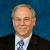# Negative Variance

Six Sigma – iSixSigma Forums Old Forums General Negative Variance

Viewing 3 posts - 1 through 3 (of 3 total)
• Author
Posts
• #44636

What is the operational definition of negative variance?

0
#143441

Jim,
There is no operational definition of a variance component that is negative. This is just the way the formula is set up (Edgeworth in the 1880s). If you get a negative variance in a variance component analysis that is possible. This is not like Analysis of Variance (by using the means) which was developed by Fisher in the 1920s. If you get a negative variance in the context of ANOVA something is wrong. I hope this helps.

0
#143472Eric Maass
Participant

Jim,
When some statistical software packages calculate Variance Components or perform Nested Analysis of Variance, or analysis that involves Nested Analysis of Variance (like Gauge Capability studies), they often do the calculations with this concept in mind:
– If there are multiple levels of nested variance, then the lower level variance can generate false variance in the higher levels
– So, to allow for this, the high level variance should be reduced by the amount of expected false variance.
– However, if the amount of expected false variance is greater than the observed high level variance, then the variance component may show up as negative…however, most programs will zero this out.
The way of estimating false variance of the higher level variance uses the Central Limit Theorem.
For example, imagine that you have lot-to-lot variance and within-lot variance. The within lot variance is 100, and the lot-to-lot is only 1. The sample size within each lot is 4.
Now, the false variance comes from this thought experiment: if you were to pretend to measure two different lots, but actually measured the same lot twice, you would see a false lot-to-lot variance. The estimate for this false lot-to-lot variance that is due to within-lot variance would be within variance/sample size from the central limit theorem. For this example, this would be 100/4 = 25.
The observed lot-to-lot variance is only 1, so the estiamte for lot-to-lot variance is the observed of 1 minus the expected false variance due to within variance of 25: 1 – 25 = -24.
Naturally, you cannot estimate the standard deviation – you’d get an imaginary number – so programs like Minitab would zero this estimate of lot-to-lot variance out.
Best regards,
Eric

0
Viewing 3 posts - 1 through 3 (of 3 total)

The forum ‘General’ is closed to new topics and replies.